# 1-Dim wave equation

Finite differences applied to the solution of a 1D wave equation PDE.
Consider the following 1D heat problem where the high function depends on space and time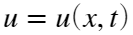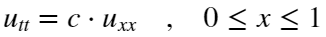with initial condition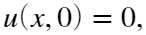and boundary conditions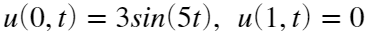.
Solve this problem for the parameter valuesThe finite differences equation are (semi-implicit method)if we definethen it can written in terms of components as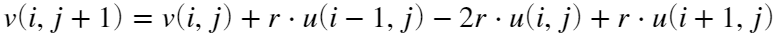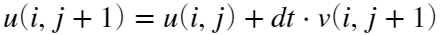clear all;
%-------------------------------------------
% Initialize variables
%-------------------------------------------
dt = 0.01; %time step
dx = 1/100; %space step
c = 0.05; %propagation coeff.
r = c/dx^2; % dt*c/dx^2; the dt term is added in the solver step
x = 0:dx:1;
m = length(x);
u = zeros(1,m);
v = zeros(1,m);
%--------------------
% Time evolution
%--------------------
numIter = 750;
t = 0; %time
for step=1:numIter
t = t+dt;
%-----------------------------------------------------
% compute acceleration values (forces) that modify velocity
%-----------------------------------------------------
for j=1:m
jleft = j-1;
if (j==1), jleft=1; end
jright = min(j+1,m);
if (j==m), jright=m; end
force(j) = r*(u(jleft)-2*u(j)+u(jright));
end
%------------------------------------
%Solver: Semi-Implicit Euler Method
%------------------------------------
v = v + dt*force; %update velocities
u = u + dt*v; %update positions with the updated velocities
%------------------------------------
u(1) = 3*sin(5*t); %impose boundary conditions;
u(end) = 0;
% -----------------------------------
plot(x, u);
axis([-0.1,1.1,-10,10]);
text(0,8,['time = ' num2str(t)]);
drawnow;
end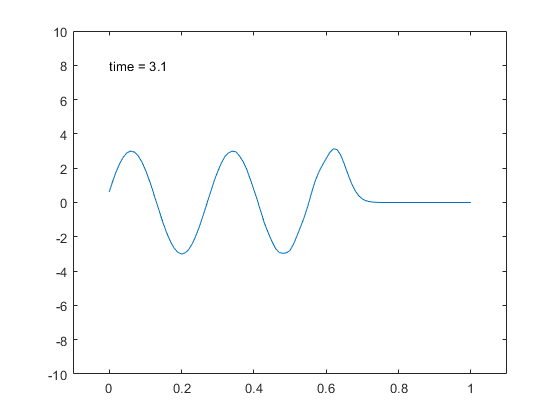Exercise 1:
Simulate the results when we consider:
initial condition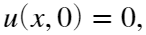and the following initial conditions to create an initial wave: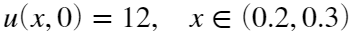and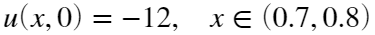boundary conditions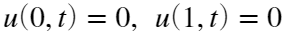.